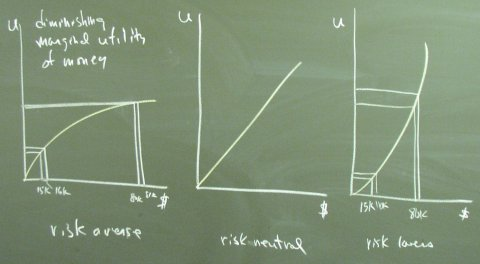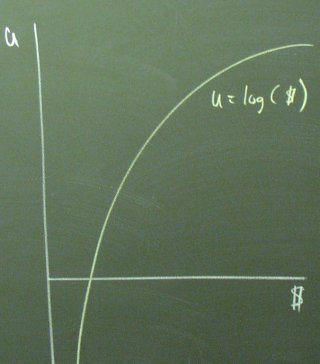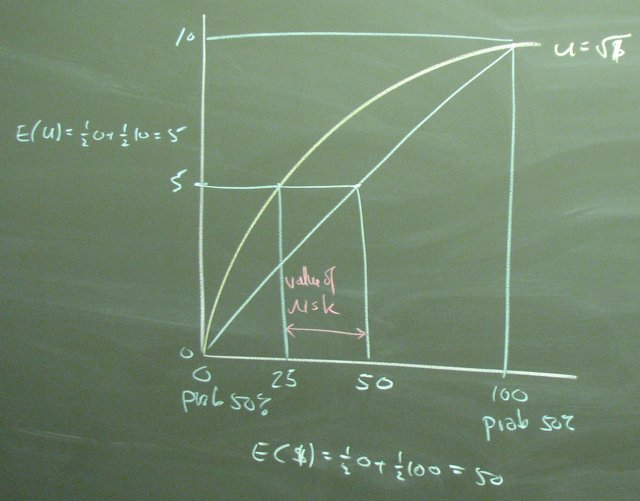February 27, 2003

Utility-Based Valuation of Risk

We first choose a utilily function type. After some explaining, we agree on the risk averse utility function, which features diminishing marginal utility of money. The square root function will be our example.The log function would also be a nice choice. It presents problems for the zero dollars and for doing lectures with easy round numbers.We use the following diagram to think about the value of the risk in a coin toss chance between 0 and 100. The risk averse agent with the curved utility function thinks it is worth 25. The risk neutral agent with the straight-line utility function thinks it is worth 50. The risk must be worth 25.The critical assumption here is that "Agents care about expected utility, not expected dollars."

Posted by bparke at February 27, 2003 02:59 PM